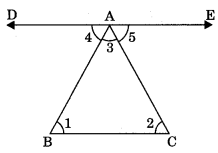On this page, you will find Lines and Angles Class 9 Notes Maths Chapter 4 Pdf free download. CBSE NCERT Class 9 Maths Notes Chapter 4 Lines and Angles will seemingly help them to revise the important concepts in less time.

## CBSE Class 9 Maths Chapter 4 Notes Lines and Angles

### Lines and Angles Class 9 Notes Understanding the Lesson

Point: A point is a dot made by a sharp pen or pencil. It is represented by capital letter.

Line: A straight and endless path on both the directions is called a line.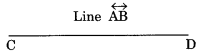Line segment: A line segment is a straight path between two points.Ray: A ray is a straight path which goes forever in one direction.Collinear points: If three or more than three points lie on the same line, then they are called collinear points.Non-collinear points: If three or more than three points does not lie on the same line, then they are called non-collinear points.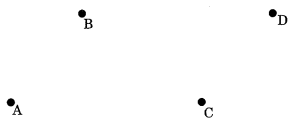Angle: The space between two straight lines that diverge from a common point or between two planes that extend from a common line.Types of Angles
1. Acute angle: An angle between 0° and 90° is called acute angle.2. Right angle: An angle which is equal to 90° is called right angle.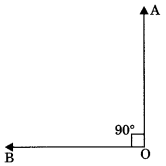3. Obtuse angle: An angle which is more than 90° but less than 180° is called obtuse angle.4. Straight angle: An angle whose measure is 180° is called straight angle.5. Reflex angle: An angle whose measure is between 180° and 360° is called reflex angle.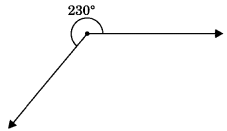6. Complete angle: An angle which is equal to 360° is called complete angle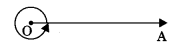Pairs of Angles

1.Complementary angles: Two angles are said to be complementary if the sum of their degree measure is 90°.For example, pair of complementary angles are 35° and 55°.

2. Supplementary angles: Two angles are said to be supplementary if the sum of their degree measure is 180°.
∠AOC + ∠BOC = 180°3. Bisector of angle: A ray which divides an angle into two equal parts is called bisector of the angle.
∠AOC = ∠BOC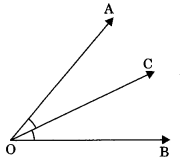4. Adjacent angles: Two angles are said to be adjacent angles if

• They have a common vertex (O)
• They have a common arm (OC)
• and their non-common arms are on either side of common arm (OA and OB).
∠AOB = ∠AOC +∠BOC

5. Linear pair: Two adjacent angles are said to be linear pair if their sum is equal to 180°.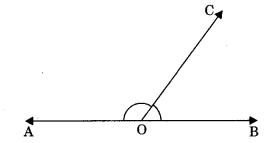∠AOC + ∠BOC = 180°
Axiom 6.1: If a ray stands on a line, then the sum of two adjacent angles so formed is 180°.
Axiom 6.2: If the sum of two adjacent angles is 180°, then the non-common arms of the angles form a line.

6. Vertically opposite angles: Vertically opposite angles are those angles which are opposite to each other (or not adjacent) when two lines cross each other.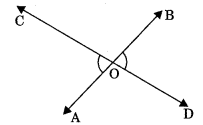Theorem 6.1: If two lines intersect each other, then the vertically opposite angles are equal.
To prove: If lines AB and CD mutually intersect at point O, then
(a) ∠AOC = ∠BOD (Vertically opposite angles)
(b) ∠AOD = ∠BOC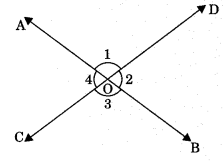Proof: Lines AB intersect CD at O.
∠1 + ∠2 = 180° (Linear pair)
∠2 + ∠3 = 180° (Linear pair)
From eqn. (1) and (2), ∠1 + ∠2 = ∠2 + ∠3
⇒ ∠1 = ∠3 ⇒ ∠AOD = ∠BOC
Similarly, ∠AOC = ∠BOD

Parallel Lines
If distance between two lines is the same at each and every point on two lines, then two lines are said to be parallel.
If lines l and m do not intersect each other at any point then l || m.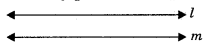Transversal line: A line is said to be transversal which intersect two or more lines at distinct points.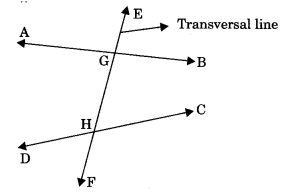1. Corresponding angles: Pair of angles having different vertex but lying on same side of the transversal are called corresponding angles. Note that in each pair one is interior and other is exterior angle.

• ∠1 and ∠2
• ∠3 and ∠4
• ∠5 and ∠6
• ∠1 and ∠8

These angles are pair of corresponding angles.2. Alternate interior angles: Pair of angles having distinct vertices and lying can either side of the transversal are called alternate interior angles.

• ∠1 and ∠2
• ∠3 and ∠4These angles are alternate interior angles

3. Consecutive interior angles: Pair of interior angles of same side of transversal line.

• ∠1 and ∠2
• ∠2 and ∠4

These angles are consecutive interior angles or co-interior angles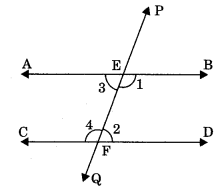Axiom 6.3: If two parallel lines are intersected by a transversal then each pair of corresponding angles are equal.
If AB || CD, then

• ∠PEB = ∠EFD
• ∠PEA = ∠EFC
• ∠BEF = ∠DFQ
• ∠AEF = ∠CFQ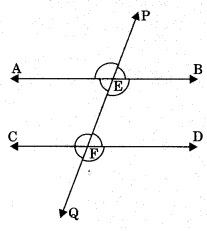Theorem 6.2: If two parallel lines are intersected by a transversal then pair of alternate interior angles are equal.
If AB || CD, then ?

• ∠AEF = ∠EFD
• ∠BEF = ∠CFE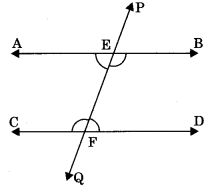Theorem 6.3: If two parallel lines are intersected by a transversal then the ! sum of consecutive interior angles of same side of transversal is equal to 180°. If AB || CD then
(i) ∠BEF + ∠DFE = 180°
(ii) ∠AEF + ∠CFE = 180°Axiom 6.4: If two lines are intersected by a transversal and a pair of corresponding angles are equal, then two lines are parallel.
(i) If ∠PEB = ∠EFD (corresponding angles), then AB || CD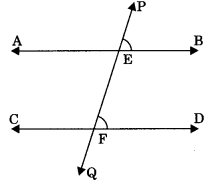Theorem 6.4: If two lines intersected by a transversal and a pair of alternate interior angles are equal, then two lines are parallel. If ∠AEF = ∠EFD (alternate interior angles), then AB || CD.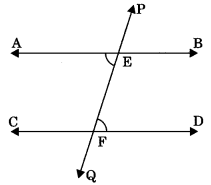Theorem 6.5: If two lines are intersected by a transversal and the sum of consecutive interior angles of same side of transversal is equal to 180°, the lines are parallel. If ∠AEF + ∠CFE = 180°, then AB || CD.Theorem 6.6: Lines which are parallel to the same line are parallel to each other.
If AB || EF and CD || EF then AB || CD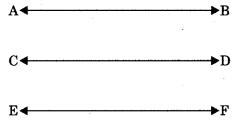Theorem 6.7: The sum of the angles of a triangle is equal to 180°.
Given: ΔABC
To prove: ∠A + ∠B + ∠C = 180°
Construction: Draw DE || BC
Proof: DE || BC
then ∠1 = ∠4 …(1) (alternate interior angles)
∠2 = ∠5 …(2) (alternate interior angles)
∠1 + ∠2 = ∠4 +∠5
∠1 +∠2 + ∠3 = ∠3 + ∠4 + ∠5
⇒ ∠A + ∠B + ∠C = 180° (Sum of angles at a point on same side of a line is 180°)

Theorem 6.8: If a side of a triangle is produced, then the exterior angle so formed is equal to the sum of the two interior opposite angles.
Given: AABC in which, side BC is produced to D.
To Prove: ∠ACD = ∠BAC + ∠ABC
Proof: ∠ACD + ∠ACB = 180° …(1) (Linear pair)
∠ABC + ∠ACB + ∠BAC = 180° …(2)
From eqn. (1) and (2), ∠ACD + ∠ACB
= ∠ABC + ∠ACB + ∠BAC
= ∠ACD = ∠ABC + ∠BAC